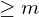# What is Competitive Programming?

Definition

Competitive Programming is a sport, which requires participants to program to solve computational problems. The score a participant gets is normally calculated based on how many problems he solved, the difficulty of the problems, the time he spends on each problem and the number of attempts he made before successfully solve each problem. Each problem is well-defined, clear and has concrete limits and constraints.

A competition can be held locally or globally. There are some annual global competitions, like IOI (International Olympiad in Informatics) – an individual-based contest for high-school students, ICPC (International Collegiate Programming Contest) – a team-based contest for college students, Google Code Jam – hosted by Google, Facebook Hacker Cup – from Facebook.

Example

Here is an example of a problem:

There are many ways to solve the above problem. I will present the one I think is the most simple:
First, we sort all the bags in the non-increasing order of the number of candies.
Then, we check if the number of candies in the biggest bag is. If it is, we output 1 as the result. If it is not, then we check if the total number of candies in the 2 biggest bags is. If it is, we output 2 as the result. If it is not, then we check the total number of candies in the 3 biggest bags and so on, until we find that the total number of candies in k biggest bags is.

Here is out solution written in C++:

#include<bits/stdc++.h>

using namespace std;

int a;
int n, m;

int main() {

cin >> n >> m;

for (int i = 1; i <= n; ++i)
cin >> a[i];

sort(a + 1, a + n + 1);
reverse(a + 1, a + n + 1);

int sum_of_candies = 0;
for (int i = 1; i <= n; ++i) {
sum_of_candies += a[i];
if (sum_of_candies >= m) {
cout << i << endl;
break;
}
}

return 0;

}


Some websites to learn and practice Competitive Programming

Codeforces

Codechef

Atcoder

Leetcode

SPOJ

Hackerrank

and many others.